# 5.1.1. EONR¶

class eonr.EONR(cost_n_fert=0.5, cost_n_social=0.0, costs_fixed=0.0, price_grain=3.0, col_n_app='rate_n_applied_lbac', col_yld='yld_grain_dry_buac', col_crop_nup='nup_total_lbac', col_n_avail='soil_plus_fert_n_lbac', col_year='year', col_location='location', col_time_n='time_n', unit_currency='$', unit_fert='lbs', unit_grain='bu', unit_area='ac', model='quad_plateau', ci_level=0.9, base_dir=None, base_zero=True, print_out=False)[source] Bases: object EONR is a Python tool for computing the optimum nitrogen rate and its confidence intervals from agricultural research data. Methods Summary  calc_delta([df_results]) Calculates the change in EONR among economic scenarios. calculate_eonr(df[, col_n_app, col_yld, …]) Calculates the EONR and its confidence intervals. plot_delta_tstat([level_list, style]) Plots the test statistic as a function nitrogen rate plot_derivative([ci_type, ci_level, style]) Plots a zoomed up view of the ONR and the derivative plot_eonr([ci_type, ci_level, run_n, x_min, …]) Plots EONR, MRTN, GRTN, net return, and nitrogen cost. plot_modify_size([fig, plotsize_x, …]) Modifies the size of the last plot generated plot_modify_title(title_text[, g, size_font]) Allows user to replace the title text plot_save([fname, base_dir, fig, dpi]) Saves a generated matplotlib figure to file plot_tau([y_axis, emphasis, run_n, style]) Plots the test statistic as a function nitrogen rate Prints the results of the optimum nitrogen rate computation set_column_names([col_n_app, col_yld, …]) Sets the column name(s) for EONR.df_data set_trial_details([year, location, n_timing]) Sets the year, location, or nitrogen timing set_units([unit_currency, unit_fert, …]) Sets the units data in EONR.df_data and for reporting update_econ([cost_n_fert, cost_n_social, …]) Sets or resets the nitrogen fertilizer cost, social cost of nitrogen, fixed costs, and/or grain price. Methods Documentation calc_delta(df_results=None)[source] Calculates the change in EONR among economic scenarios. EONR.calc_delta filters all data by location, year, and nitrogen timing, then the “delta” is calculated as the difference relative to the economic scenario resulting in the highest EONR. Parameters df_results (Pandas dataframe, optional) – The dataframe containing the results from EONR.calculate_eonr() (default: None). Returns df_delta – The dataframe with the newly inserted EONR delta. Return type pandas.DataFrame Example Please complete the EONR.calculate_eonr example first because this example builds on the results of the my_eonr object. Change the economic scenario (using EONR.calculate_eonr) and calculate the EONR again for the same dataset (using EONR.calculate_eonr) >>> price_grain = 0.314 # in USD per kg grain >>> my_eonr.update_econ(price_grain=price_grain) >>> my_eonr.calculate_eonr(df_data) Computing EONR for Minnesota 2012 Pre Cost of N fertilizer:$0.88 per kg
Price grain: $0.31 per kg Fixed costs:$0.00 per ha
Economic optimum N rate (EONR): 169.9 kg per ha [135.2, 220.9] (90.0% confidence)
Maximum return to N (MRTN): $1682.04 per ha  Use EONR.calc_delta to >>> df_delta = my_eonr.calc_delta(my_eonr.df_results)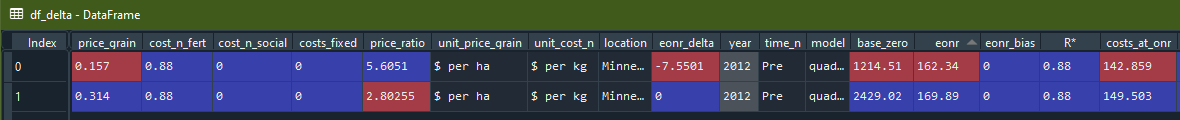calculate_eonr(df, col_n_app=None, col_yld=None, col_crop_nup=None, col_n_avail=None, col_year=None, col_location=None, col_time_n=None, bootstrap_ci=False, samples_boot=9999, delta_tstat=False)[source] Calculates the EONR and its confidence intervals. col_n_app and col_yld are required by EONR, but not necessarily by EONR.calculate_eonr(). They must either be set during the initialization of EONR, before running EONR.calculate_eonr (using EONR.set_column_names), or they must be passed in this EONR.calculate_eonr method. Parameters • df (Pandas dataframe) – The dataframe containing the experimental data. • col_n_app (str, optional) – Column name pointing to the rate of applied N fertilizer data (default: None). • col_yld (str, optional) – Column name pointing to the grain yield data. This column is multiplied by price_grain to create the ‘grtn’ column in EONR.df_data (default: None). • col_crop_nup (str, optional) – Column name pointing to crop N uptake data (default: None). • col_n_avail (str, optional) – Column name pointing to available soil N at planting plus fertilizer throughout the season (default: None). • col_year (str, optional) – Column name pointing to year (default: None). • col_location (str, optional) – Column name pointing to location (default: None). • col_time_n (str, optional) – Column name pointing to nitrogen application timing (default: None). • bootstrap_ci (bool, optional) – Indicates whether bootstrap confidence intervals are to be computed. If calculating the EONR for many sites and/or economic scenarios, it may be desirable to set to False because the bootstrap confidence intervals take the most time to compute (default: False). • samples_boot (int, optional) – Number of samples in the bootstrap computation (default: 9999). • delta_tstat (bool, optional) – Indicates whether the difference from the t-statistic will be computed (as a function of theta2/N rate). May be useful to observe what optimization method is best suited to reach convergence when computing the profile-likelihood CIs (default: False). Note col_crop_nup and col_n_avail are required to calculate the socially optimum nitrogen rate, SONR. The SONR is the optimum nitrogen rate considering the social cost of nitrogen, so therefore, EONR.cost_n_social must also be set. col_year, col_location, and col_time_n are purely optional. They only affect the titles and axes labels of the plots. Example Load and initialize eonr >>> from eonr import EONR >>> import os >>> import pandas as pd  Load the sample data >>> base_dir = r'F:\nigo0024\Documents\GitHub\eonr\eonr' >>> df_data = pd.read_csv(os.path.join(base_dir, 'data', 'minnesota_2012.csv'))  Set column names >>> col_n_app = 'rate_n_applied_kgha' >>> col_yld = 'yld_grain_dry_kgha'  Set units >>> unit_currency = '$'
>>> unit_fert = 'kg'
>>> unit_grain = 'kg'
>>> unit_area = 'ha'


Set economic conditions

>>> cost_n_fert = 0.88  # in USD per kg nitrogen
>>> price_grain = 0.157  # in USD per kg grain


Initialize EONR

>>> my_eonr = EONR(cost_n_fert=cost_n_fert,
price_grain=price_grain,
col_n_app=col_n_app,
col_yld=col_yld,
unit_currency=unit_currency,
unit_grain=unit_grain,
unit_fert=unit_fert,
unit_area=unit_area,
model=None,
base_dir=base_dir)


Calculate the economic optimum nitrogen rate using EONR.calculate_eonr

>>> my_eonr.calculate_eonr(df_data)
Computing EONR for Minnesota 2012 Pre
Cost of N fertilizer: $0.88 per kg Price grain:$0.16 per kg
Fixed costs: $0.00 per ha Checking quadratic and quadric-plateau models for best fit.. Quadratic model r^2: 0.72 Quadratic-plateau model r^2: 0.73 Using the quadratic-plateau model.. Economic optimum N rate (EONR): 162.3 kg per ha [130.5, 207.8] (90.0% confidence) Maximum return to N (MRTN):$767.93 per ha

plot_delta_tstat(level_list=None, style='ggplot')[source]

Plots the test statistic as a function nitrogen rate

Parameters
• level_list (list) – The confidence levels to plot; should be a subset of items in EONR.ci_list (default: None).

• style (str, optional) – The style of the plolt; can be any of the options supported by matplotlib

Example

Load and initialize eonr, then load the sample data

>>> from eonr import EONR
>>> import os
>>> import pandas as pd
>>> base_dir = r'F:\nigo0024\Documents\GitHub\eonr\eonr'
>>> df_data = pd.read_csv(os.path.join(base_dir, 'data', 'minnesota_2012.csv'))


Set column names, units, and economic conditions

>>> col_n_app = 'rate_n_applied_kgha'
>>> col_yld = 'yld_grain_dry_kgha'
>>> unit_currency = '$' >>> unit_fert = 'kg' >>> unit_grain = 'kg' >>> unit_area = 'ha' >>> cost_n_fert = 0.88 # in USD per kg nitrogen >>> price_grain = 0.157 # in USD per kg grain  Initialize EONR >>> my_eonr = EONR(cost_n_fert=cost_n_fert, price_grain=price_grain, col_n_app=col_n_app, col_yld=col_yld, unit_currency=unit_currency, unit_grain=unit_grain, unit_fert=unit_fert, unit_area=unit_area, model=None, base_dir=base_dir)  Calculate the economic optimum nitrogen rate using EONR.calculate_eonr, being sure to set delta_stat to True >>> my_eonr.calculate_eonr(df_data, delta_tstat=True) Computing EONR for Minnesota 2012 Pre Cost of N fertilizer:$0.88 per kg
Price grain: $0.16 per kg Fixed costs:$0.00 per ha
Economic optimum N rate (EONR): 162.3 kg per ha [130.5, 207.8] (90.0% confidence)
Maximum return to N (MRTN): $767.93 per ha  Plot the Delta t-stat plot using EONR.plot_delta_tstat >>> my_eonr.plot_delta_tstat()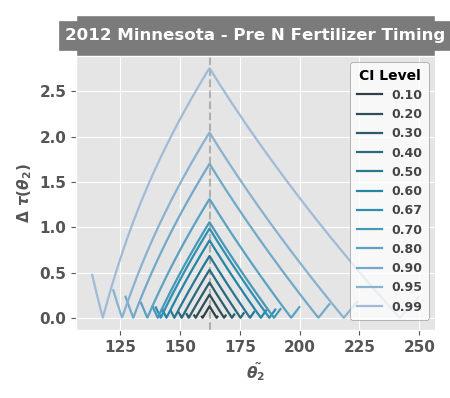plot_derivative(ci_type='profile-likelihood', ci_level=None, style='ggplot')[source] Plots a zoomed up view of the ONR and the derivative Parameters • ci_type (str) – Indicates which confidence interval type should be plotted. Options are ‘wald’, to plot the Wald CIs; ‘profile-likelihood’, to plot the profile-likelihood CIs; or ‘bootstrap’, to plot the bootstrap CIs (default: ‘profile-likelihood’). • ci_level (float) – The confidence interval level to be plotted, and must be one of the values in EONR.ci_list. If None, uses the EONR.ci_level (default: None). • level (float) – The confidence levels to plot; should be a value from EONR.ci_list (default: 0.90). • style (str, optional) – The style of the plolt; can be any of the options supported by matplotlib Example Please complete the EONR.calculate_eonr example first because this example builds on the results of the my_eonr object. >>> my_eonr.plot_derivative()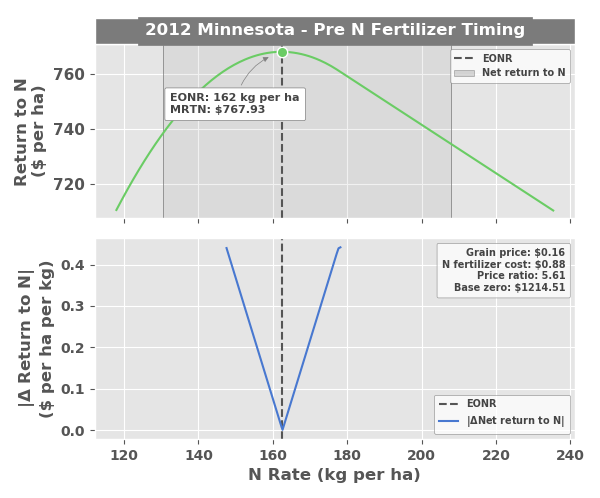plot_eonr(ci_type='profile-likelihood', ci_level=None, run_n=None, x_min=None, x_max=None, y_min=None, y_max=None, show_model=True, style='ggplot')[source] Plots EONR, MRTN, GRTN, net return, and nitrogen cost. If left as None, x_min, x_max, y_min, and y_max are set by Matplotlib. Parameters • ci_type (str, optional) – Indicates which confidence interval type should be plotted. Options are ‘wald’, to plot the Wald CIs; ‘profile-likelihood’, to plot the profile-likelihood CIs; or ‘bootstrap’, to plot the bootstrap CIs (default: ‘profile-likelihood’). • ci_level (float, optional) – The confidence interval level to be plotted, and must be one of the values in EONR.ci_list. If None, uses the EONR.ci_level (default: None). • run_n (int, optional) – NOT IMPLEMENTED. The run number to plot, as indicated in EONR.df_results; if None, uses the most recent, or maximum, run_n in EONR.df_results (default: None). • x_min (int, optional) – The minimum x-bounds of the plot (default: None) • x_max (int, optional) – The maximum x-bounds of the plot (default: None) • y_min (int, optional) – The minimum y-bounds of the plot (default: None) • y_max (int, optional) – The maximum y-bounds of the plot (default: None) • show_model (str) – Whether to display the type of fitted model in the helper legend (default: True). • style (str, optional) – The style of the plot; can be any of the options supported by matplotlib (default: ‘ggplot’). Example Please complete the EONR.calculate_eonr example first because this example builds on the results of the my_eonr object. >>> my_eonr.plot_eonr(x_min=-5, x_max=300, y_min=-100, y_max=1400)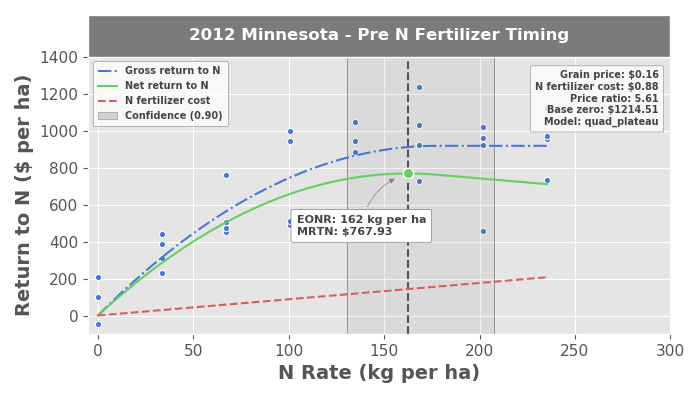plot_modify_size(fig=None, plotsize_x=7, plotsize_y=4, labelsize=11)[source] Modifies the size of the last plot generated Parameters • fig (Matplotlib Figure, optional) – Matplotlib figure to modify (default: None) • plotsize_x (float, optional) – Sets x size of plot in inches (default: 7) • plotsize_y (float, optional) – Sets y size of plot in inches (default: 4) • labelsize (float, optional) – Sets tick and label (defaulat: 11) Example Please complete the EONR.calculate_eonr and EONR.plot_eonr examples first because this example builds on the results of the my_eonr.fig_eonr.fig object. >>> my_eonr.plot_modify_size(fig=my_eonr.fig_eonr.fig, plotsize_x=5, plotsize_y=3, labelsize=9)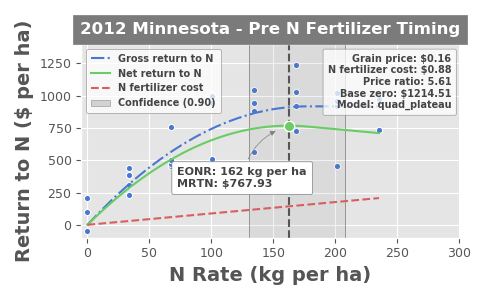plot_modify_title(title_text, g=None, size_font=12)[source] Allows user to replace the title text Parameters • title_text (str) – New title text • g (matplotlib.figure) – Matplotlib figure object to modify (default: None) • size_font (float) – Font size to use (default: 12) Example Please complete the EONR.calculate_eonr and EONR.plot_eonr examples first because this example builds on the results of the my_eonr.fig_eonr.fig object. >>> my_eonr.plot_modify_title('Preplant N fertilizer - Stewart, MN 2012', g=my_eonr.fig_eonr.fig, size_font=15)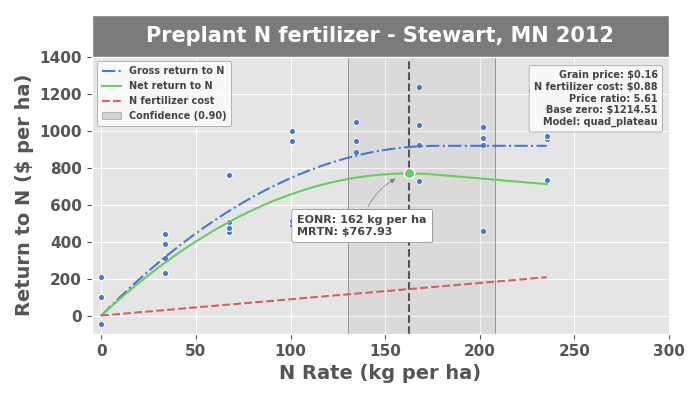plot_save(fname=None, base_dir=None, fig=None, dpi=300)[source] Saves a generated matplotlib figure to file Parameters • fname (str, optional) – Filename to save plot to (default: None) • base_dir (str, optional) – Base file directory when saving results (default: None) • fig (eonr.fig, optional) – EONR figure object to save (default: None) • dpi (int, optional) – Resolution to save the figure to in dots per inch (default: 300) Example Please complete the EONR.calculate_eonr and EONR.plot_eonr examples first because this example builds on the results of the my_eonr.fig_eonr.fig object. Set output filename >>> fname = r'F:\nigo0024\Downloads\eonr_fig.png'  Save the most recent figure >>> my_eonr.plot_save(fname) fig is None, so saving the current (most recent) figure.  >>> os.path.isfile(fname) True  plot_tau(y_axis='t_stat', emphasis='profile-likelihood', run_n=None, style='ggplot')[source] Plots the test statistic as a function nitrogen rate Parameters • y_axis (str, optional) – Value to plot on the y-axis. Options are ‘t_stat’, to plot the T statistic; ‘f_stat’, to plot the F-statistic; or ‘level’, to plot the confidence level; (default: ‘t_stat’). • emphasis (str, optional) – Indicates which confidence interval type, if any, should be emphasized. Options are ‘wald’, to empahsize the Wald CIs; ‘profile-likelihood’, to empahsize the profile-likelihood CIs; ‘bootstrap’, to empahsize the bootstrap CIs; or None, to empahsize no CI (default: ‘profile-likelihood’). • run_n (int, optional) – The run number to plot, as indicated in EONR.df_ci; if None, uses the most recent, or maximum, run_n in EONR.df_ci (default: None). • style (str, optional) – The style of the plolt; can be any of the options supported by matplotlib Example Please complete the EONR.calculate_eonr example first because this example builds on the results of the my_eonr object. >>> my_eonr.plot_tau()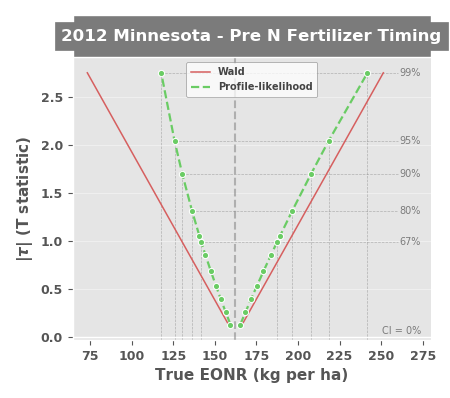print_results()[source] Prints the results of the optimum nitrogen rate computation Example Please complete the EONR.calculate_eonr example first because this example builds on the results of the my_eonr object. >>> my_eonr.print_results() Economic optimum N rate (EONR): 162.3 kg per ha [130.5, 207.8] (90.0% confidence) Maximum return to N (MRTN):$767.93 per ha

set_column_names(col_n_app=None, col_yld=None, col_crop_nup=None, col_n_avail=None, col_year=None, col_location=None, col_time_n=None)[source]

Sets the column name(s) for EONR.df_data

If these descriptions are used as metadata in the input dataset, they are accessed for plotting purposes. These parameters do not affect the calculation of the EONR or its confidence intervals in any way.

Parameters
• col_n_app (str, optional) – Column name pointing to the rate of applied N fertilizer data (default: None).

• col_yld (str, optional) – Column name pointing to the grain yield data. This column is multiplied by price_grain to create the ‘grtn’ column in EONR.df_data (default: None).

• col_crop_nup (str, optional) – Column name pointing to crop N uptake data (default: None).

• col_n_avail (str, optional) – Column name pointing to available soil N at planting plus fertilizer throughout the season (default: None).

• col_year (str, optional) – Column name pointing to year (default: None).

• col_location (str, optional) – Column name pointing to location (default: None).

• col_time_n (str, optional) – Column name pointing to nitrogen application timing (default: None).

Example

Load and initialize eonr

>>> from eonr import EONR
>>> import os
>>> import pandas as pd
>>> base_dir = r'F:\nigo0024\Documents\GitHub\eonr\eonr'
>>> my_eonr = EONR(model=None, base_dir=base_dir)


Set the column names using EONR.set_column_names

>>> my_eonr.set_column_names(col_n_app='rate_n_applied_kgha', col_yld='yld_grain_dry_kgha')
>>> print(my_eonr.col_n_app)
>>> print(my_eonr.col_yld)
rate_n_applied_kgha
yld_grain_dry_kgha

set_trial_details(year=None, location=None, n_timing=None)[source]

Sets the year, location, or nitrogen timing

If these descriptions are used as metadata in the input dataset, they are accessed for plotting purposes. These parameters do not affect the calculation of the EONR or its confidence intervals in any way.

Parameters
• year (str or int, optional) – Year of experimental trial (default: None)

• location (str or int, optional) – Location of experimental trial (default: None)

• n_timing (str or int, optional) – Nitrogen timing of experimental trial (default: None)

Example

Load and initialize eonr

>>> from eonr import EONR
>>> import os
>>> import pandas as pd
>>> base_dir = r'F:\nigo0024\Documents\GitHub\eonr\eonr'
>>> my_eonr = EONR(model=None, base_dir=base_dir)


Set the trial details using EONR.set_trial_details

>>> my_eonr.set_trial_details(year=2019, location='St. Paul, MN', n_timing='At planting')
>>> print(my_eonr.year)
>>> print(my_eonr.location)
>>> print(my_eonr.n_timing)
2019
St. Paul, MN
At planting

set_units(unit_currency=None, unit_fert=None, unit_grain=None, unit_area=None)[source]

Sets the units data in EONR.df_data and for reporting

Parameters
• unit_currency (str, optional) – Currency unit, e.g., “\$” (default: None).

• unit_fert (str, optional) – Fertilizer unit, e.g., “lbs” (default: None).

• unit_grain (str, optional) – Grain unit, e.g., “bu” (default: None).

• unit_area (str, optional) – Area unit, e.g., “ac” (default: None).

Example

Load and initialize eonr

>>> from eonr import EONR
>>> import os
>>> import pandas as pd
>>> base_dir = r'F:\nigo0024\Documents\GitHub\eonr\eonr'
>>> my_eonr = EONR(model=None, base_dir=base_dir)


Set the units using EONR.set_units

>>> my_eonr.set_units(unit_currency='USD', unit_fert='kg', unit_grain='kg', unit_area='ha')
>>> print(my_eonr.unit_currency)
>>> print(my_eonr.unit_fert)
>>> print(my_eonr.unit_grain)
>>> print(my_eonr.unit_area)
USD
kg
kg
ha

update_econ(cost_n_fert=None, cost_n_social=None, costs_fixed=None, price_grain=None)[source]

Sets or resets the nitrogen fertilizer cost, social cost of nitrogen, fixed costs, and/or grain price.

The price ratio is recomputed based on the passed information, then the the lowest level folder in the base directory is renamed/adjusted (EONR.base_dir) based on to the price ratio. The folder name is set according to the economic scenario (useful when running EONR for many different economic scenarios then plotting and saving results for each scenario).

Parameters
• cost_n_fert (float, optional) – Cost of nitrogen fertilizer (default: None).

• cost_n_social (float, optional) – Cost of pollution caused by excess nitrogen (default: None).

• costs_fixed (float, optional) – Fixed costs on a per area basis

• (default – None)

• price_grain (float, optional) – Price of grain (default: None).

Example

Load and initialize eonr

>>> from eonr import EONR
>>> import os
>>> import pandas as pd
>>> base_dir = r'F:\nigo0024\Documents\GitHub\eonr\eonr'
>>> my_eonr = EONR(model=None, base_dir=base_dir)


Set/update the cost of fertilizer and price of grain using EONR.update_econ

>>> my_eonr.update_econ(cost_n_fert=0.88, price_grain=0.157)
>>> print(my_eonr.price_ratio)
>>> print(my_eonr.base_dir)
5.605095541

Set/update the social cost of nitrogen, again using EONR.update_econ
>>> my_eonr.update_econ(cost_n_social=1.1)